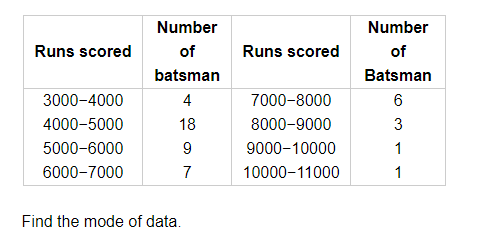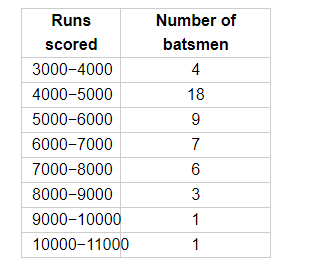# The given distribution shows the number of runs scored by`
Question:

The given distribution shows the number of runs scored by some top batsmen of the world in one-day international cricket matches.Solution:

The given data is shown below.Here, the maximum frequency is 18 so the modal class is 4000-5000.

Therefore,

$l=4000$

$h=1000$

$f=18$

$f_{1}=4$

$f_{2}=9$

$\therefore$ Mode $=l+\frac{f-f_{1}}{2 f-f_{1}-f_{2}} \times h$

$=4000+\frac{18-4}{2 \times 18-4-9} \times 1000$

$=4000+\frac{14}{23} \times 1000$

$=4000+608.7$

$=4608.7$

Thus, the mode of the data (or runs scored) is 4608.7.